## Sunday, June 2, 2013

### HP 35S: Planar Triangles

This program is set to solve common problems in plane (regular) triangles.

The programs can be adapted to any side lengths and angles necessary.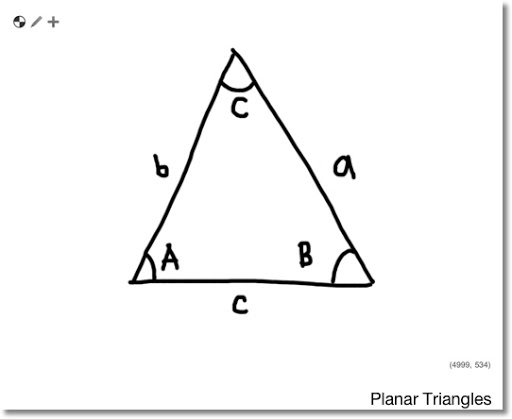Variables of Plane Triangles

Side length a with corresponding angle A,
Side length b with corresponding angle B, and
Side length c with corresponding angle C.

Labels and Stack Set Up: HP 35S

Angle-Angle-Side, Label P001, Stack: B, A, b, Goal: a

Side-Side-Angle, Label P009, Stack: b, a, B, Goal: A

Angle-Side-Angle, Label P015, Stack: b, A, c, Goal: a

Side-Side-Side, Label P033, Stack: a, b, c, Goal: A (angle corresponding to first side length entered)

* If you use a 15C, 32Sii, or other another RPN calculator, you will need to create four labels. The nice thing with the HP 35S is that you can create multiple programs within in one label. Memory registers B and C are temporary.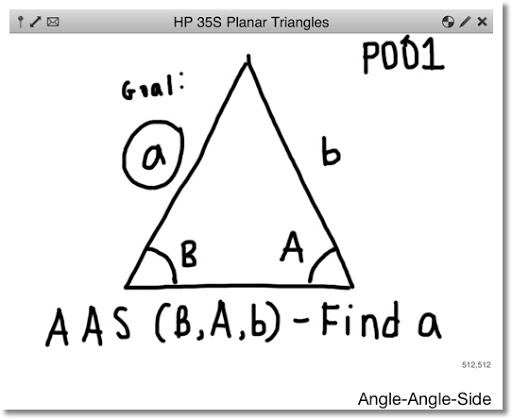Program P (Planar Triangles)

\\ Angle-Angle-Side: Law of Sines
\\ Stack: B, A, b; Find: a

P001 LBL P
P002 x<>y
P003 SIN
P004 ×
P005 x<>y
P006 SIN
P007 ÷
P008 RTN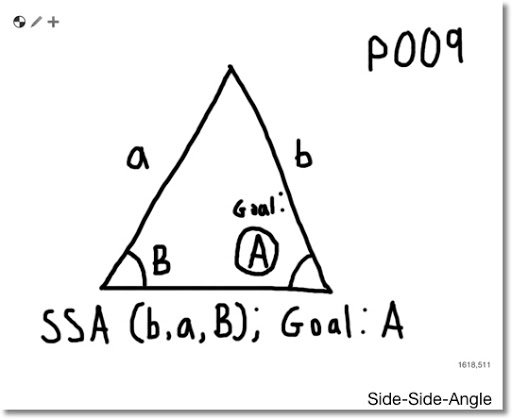\\ Side-Side-Angle: Law of Sines
\\ Stack: b, a, B; Find: A
P009 SIN
P010 ×
P011 x<>y
P012 ÷
P013 ASIN
P014 RTN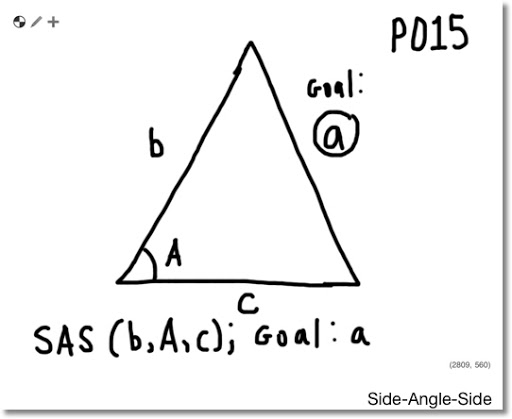\\ Side-Angle-Side: Law of Cosines
\\ Stack: b, A, c; Find: a
P015 STO C
P016 x<>y
P017 COS
P018 ×
P019 x<>y
P020 STO B
P021 ×
P022 2
P023 ×
P024 +/-
P025 RCL B
P026 x^2
P027 +
P028 RCL C
P029 x^2
P030 +
P031 √
P032 RTN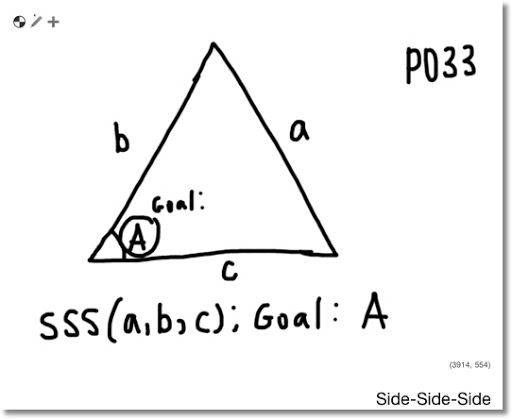\\ Side-Side-Side: Law of Cosines
\\ Stack: a, b, c; Find: A
P033 STO C
P034 x^2
P035 x<>y
P036 STO B
P037 x^2
P038 +
P039 x<>y
P040 x^2
P041 -
P042 2
P043 ÷
P044 RCL÷ B
P045 RCL÷ C
P046 ACOS
P047 RTN

Examples (Degrees Mode Used):
AAS: B = 30, A = 40, b = 4; a ≈ 5.1423

SSA: b = 5, a = 4, B = 90°; A ≈ 53.1301°

SAS: b = 8, A= 30°, c = 9; a ≈ 4.5047

SSS: a = 5, b = 4, c = 3; A = 90°

Hope this helps. I plan to post a program regarding spherical triangles.

Take care,

Eddie

This blog is property of Edward Shore. 2013

#### 1 comment:

1.This comment has been removed by the author.

### Retro Review: TI-95 Procalc

Retro Review:  TI-95 Procalc  This review covers the calculator itself, not any of the optional accessories which includes a ...